# Meter Definition

Amberflo provides a comprehensive set of Meter Types that allow for the tracking and aggregation of any resource. We aim to provide a flexible set of options for the frequency and method of reporting (usage events).

At a high level, we have separated the meter creation process into three stages. In the first, you select the meter type. This includes the type of meter event, the type of meter value that will be sent (reporting method), the usage metric to be applied to the data, and the timeout interval.

Select a meter type based on the data type and tracking method. Amberflo Cloud Metering Service automatically collects, persists, and aggregates the meter data in real-time. Meters are defined based on the Usage Metric and the Pipeline that are selected as shown in the meter list view below.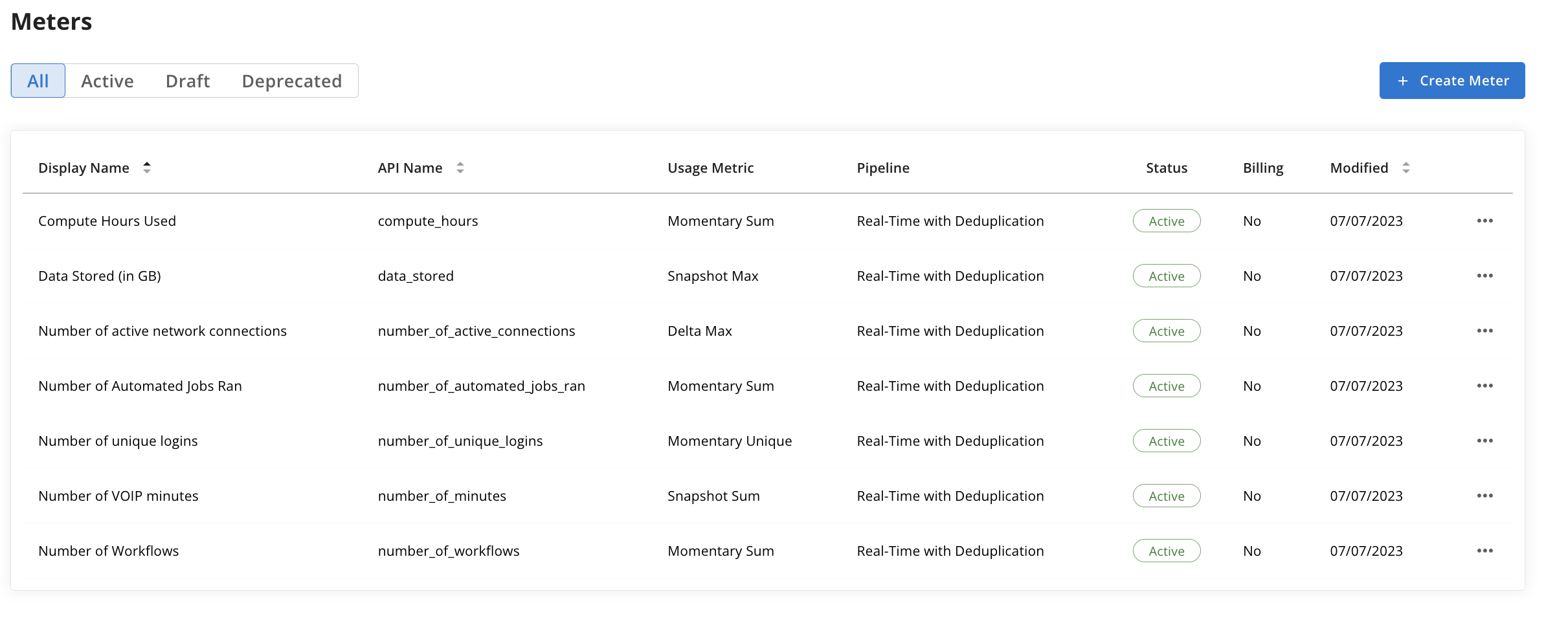# Momentary Events

Momentary events track the number of times that a completed action has taken place. Example resources tracked by this meter type include API calls, read calls, button clicks, and active user tracking.

## Sum of all usage

Report a number or amount at a distinct time. Amberflo will compute the total of all values reported within a selected time interval per customer.

This meter is created by selecting Momentary as the type of meter event and Sum as the usage metric.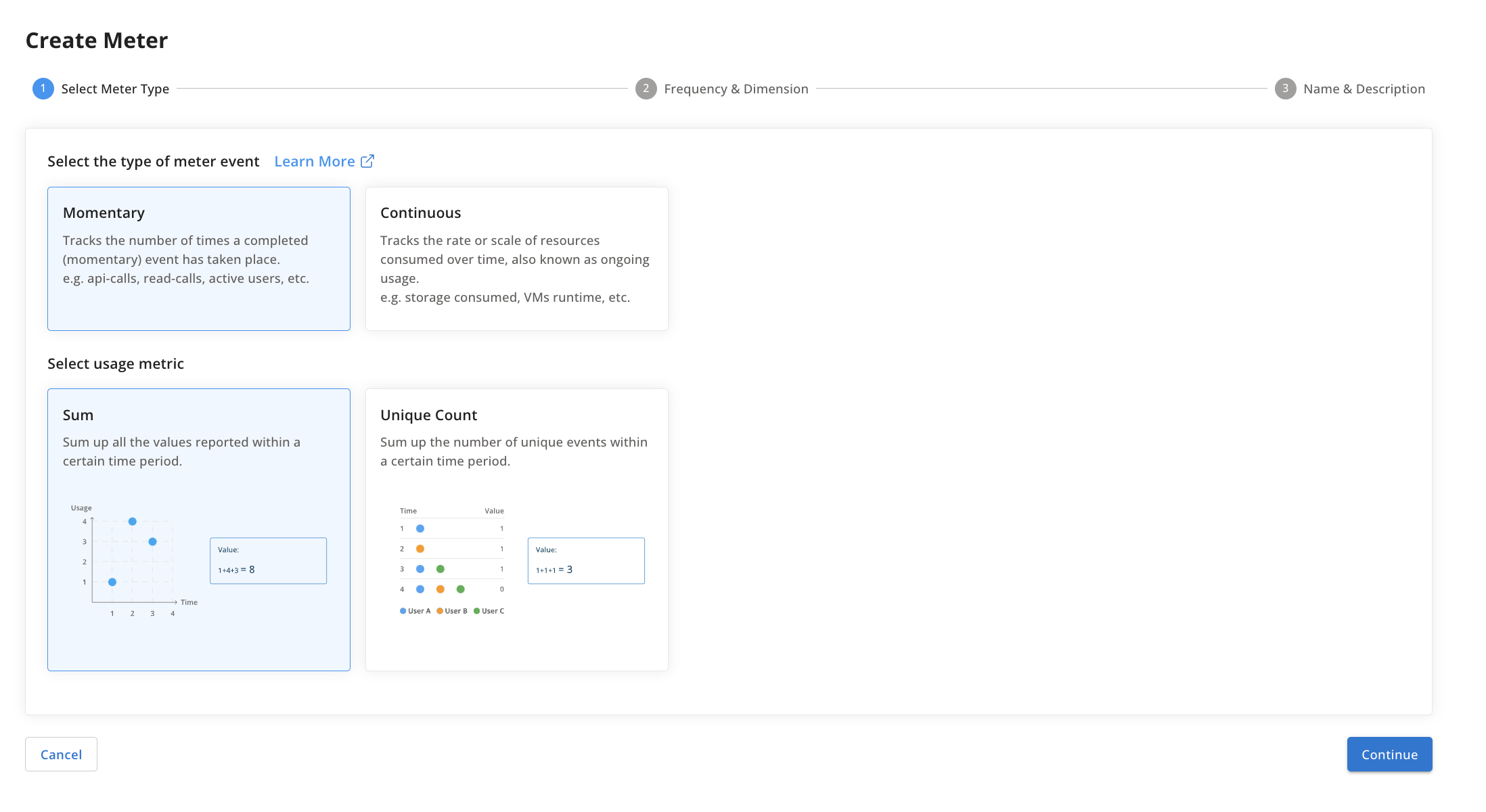Resource
A dynamic resource is consumed by the customer momentarily. Examples of such resources are API calls, bytes uploaded or downloaded, reads, writes, etc.

Meter Calculation
This meter tracks and provides the usage events of such a resource by the customer.

Meter Value
The incremental change since the previous ingestion event (aka delta).

Aggregation: sum

📘

Use Cases

A meter called API Calls reports a value of 1 every time the API endpoint is called. For any given time interval, you want to know how many API Calls were made during that interval.

A meter called Files Opened reports a value of 1 every time a file is opened. For any given time interval, show the total number of files were opened.

A meter called Bytes_Written reports a value of [the number of bytes written] every time a Write operation is executed. For any given time interval, show the total number of Bytes written.

Other examples: bytes read, bytes written, data volume, projects.

👍

Example Calculation

Given:

• Meter Definition created with name: ApiCalls. In this example, the meter is ingested at real time whenever a call is made to an API.

When the following events are created:

• <time(day 1, 1:10), meterName(ApiCalls), customer(Stark), value(1)>
• <time(day 1, 1:15), meterName(ApiCalls), customer(Stark), value(1)>
• <time(day 1, 1:45), meterName(ApiCalls), customer(Stark), value(1)>
• <time(day 1, 1:45), meterName(ApiCalls), customer(Wayne), value(1)>
• <time(day 1, 1:55), meterName(ApiCalls), customer(Stark), value(1)>
• <time(day 2, 1:00), meterName(ApiCalls), customer(Stark), value(1)>
• <time(day 2, 9:00), meterName(ApiCalls), customer(Stark), value(1)>
• <time(day 3, 1:15), meterName(ApiCalls), customer(Stark), value(1)>
• <time(day 3, 3:45), meterName(ApiCalls), customer(Stark), value(1)>
• <time(day 4, 1:45), meterName(ApiCalls), customer(Wayne), value(1)>
• <time(day 4, 23:30), meterName(ApiCalls), customer(Stark), value(1)>

Then:

• Query range of day 1 to day 1 for customer Stark -> value 4
• Query range of day 1 to day 1 for customer Wayne -> value 1
• Query range of day 2 to day 2 for customer Stark -> value 2
• Query range of day 3 to day 3 for customer Stark -> value 2
• Query range of day 1 to day 3 for customer Stark -> value 8
• Query range of day 1 to day 3 for all customers -> value 9
• Query range of day 4 to day 4 for customer Stark -> value 1
• Query range of day 4 to day 4 for all customers -> value 2

## Unique Count

This type of meter tracks the number of unique (completed) events to take place within a given time interval. Event uniqueness is defined by the uniqueDimension parameter.

Amberflo will compute the number of unique events within the time interval. This meter only accepts a value of 1 since it is mainly tracking the occurrence of an event.

This meter is created by selecting Momentary as the type of meter event and Unique Count as the usage metric.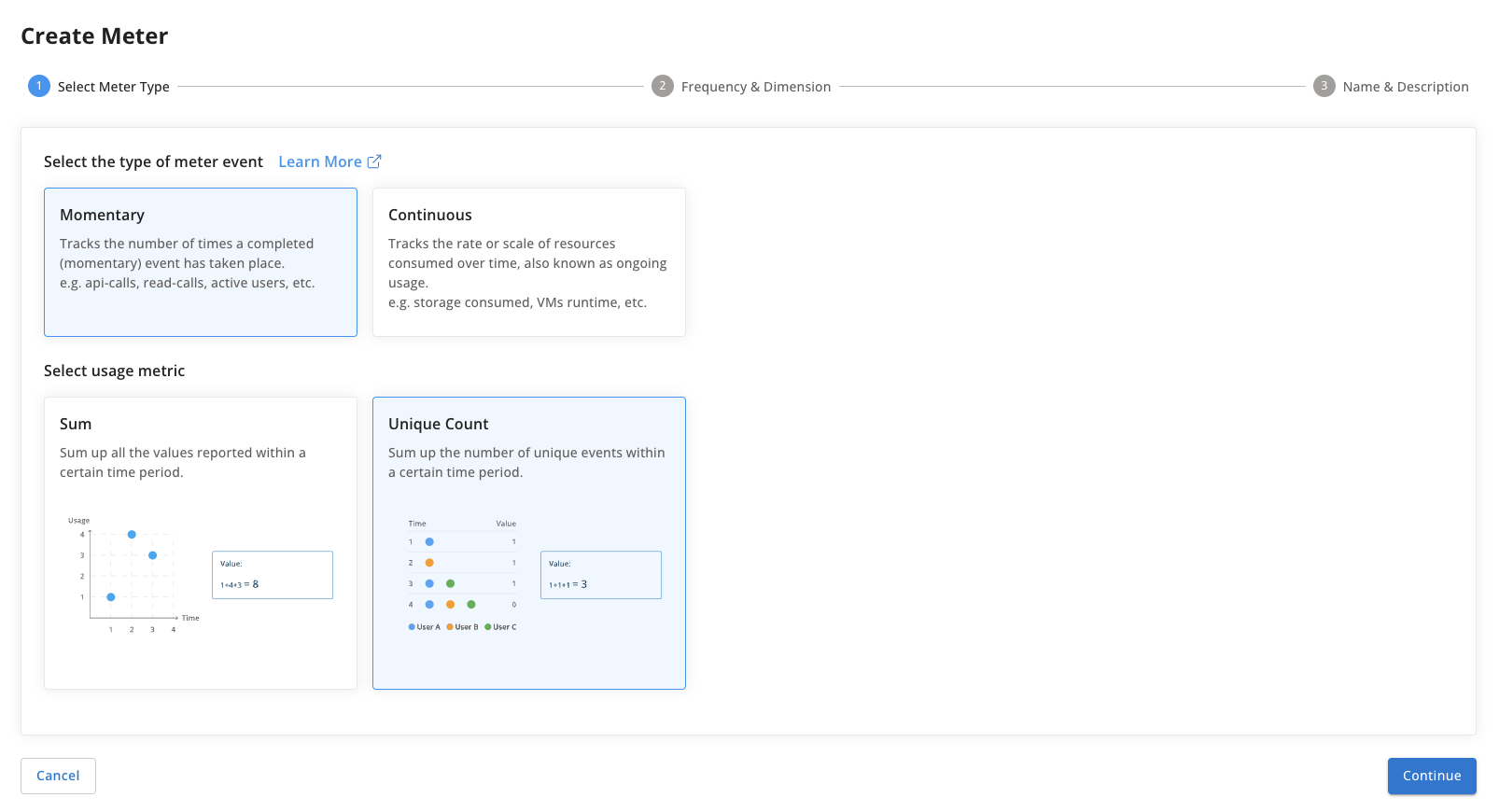Resource
A static resource is assigned to a customer. Examples: VMs used, workflow count, user login, etc.

Meter Calculation
This meter tracks the scale or frequency a resource is being assigned to a customer over time. In other words, the meter tracks the distinct usage rate.

Meter Value
This meter can only accept a value of 1 since it is mainly tracking the occurrence of an event.

Aggregation: distinct max

📘

Example

Other examples: user logins, page likes.

👍

Example Calculation

Given:

• Meter Definition created with name: UniqueUserLogins and uniqueDimension: userId

When the following events are created:

• <time(day 1, 1:10), meterName(UniqueUserLogins), customer(Wayne), userId(batman), value(1)>
• <time(day 1, 1:15), meterName(UniqueUserLogins), customer(Wayne), userId(robin), value(1)>
• <time(day 1, 1:45), meterName(UniqueUserLogins), customer(Wayne), userId(joker), value(1)>
• <time(day 1, 1:55), meterName(UniqueUserLogins), customer(Wayne), userId(batman), value(1)>
• <time(day 2, 1:00), meterName(UniqueUserLogins), customer(Wayne), userId(joker), value(1)>
• <time(day 2, 9:00), meterName(UniqueUserLogins), customer(Wayne), userId(robin), value(1)>
• <time(day 3, 1:15), meterName(UniqueUserLogins), customer(Wayne), userId(batman), value(1)>
• <time(day 3, 3:45), meterName(UniqueUserLogins), customer(Wayne), userId(batman), value(1)>
• <time(day 4, 23:30), meterName(UniqueUserLogins), customer(Wayne), userId(robin), value(1)>

Then:

• Query range of day 1 to day 1 -> value 3
• Query range of day 2 to day 2 -> value 2
• Query range of day 3 to day 3 -> value 1
• Query range of day 1 to day 3 -> value 3
• Query range of day 4 to day 4 -> value 1

# Continuous Events

This meter type tracks the usage rate or scale of ongoing usage that takes place over time. To calculate true usage from the usage rate, one must calculate the integral/area under the curve that shows usage rate over time. Example resources tracked by this meter type include data storage and VM runtime.

### Selecting the type of meter value - Snapshot vs Delta

Reporting meter values as snapshots is the recommended practice. Using this approach, the meter value is equal to the total usage rate at that time.

When using the Delta approach, the meter value is equal to the difference between the previous usage rate and the current usage rate. Said another way, it is the magnitude of the change from the previous event to the current time.

# Snapshot Meters

## Event Duration

Duration is tracked using the eventId dimension. A start event with value 1 is sent when usage begins. The count remains active until a new meter event decrements it (ie. a stop event having the same eventId dimension with value 0). The value of 1 is carried over to the next hour until the event is ended or the timeout is reached.

This meter is created by selecting Continuous as the type of meter event, Snapshot as the type of meter value that will be sent, and Sum as the usage metric.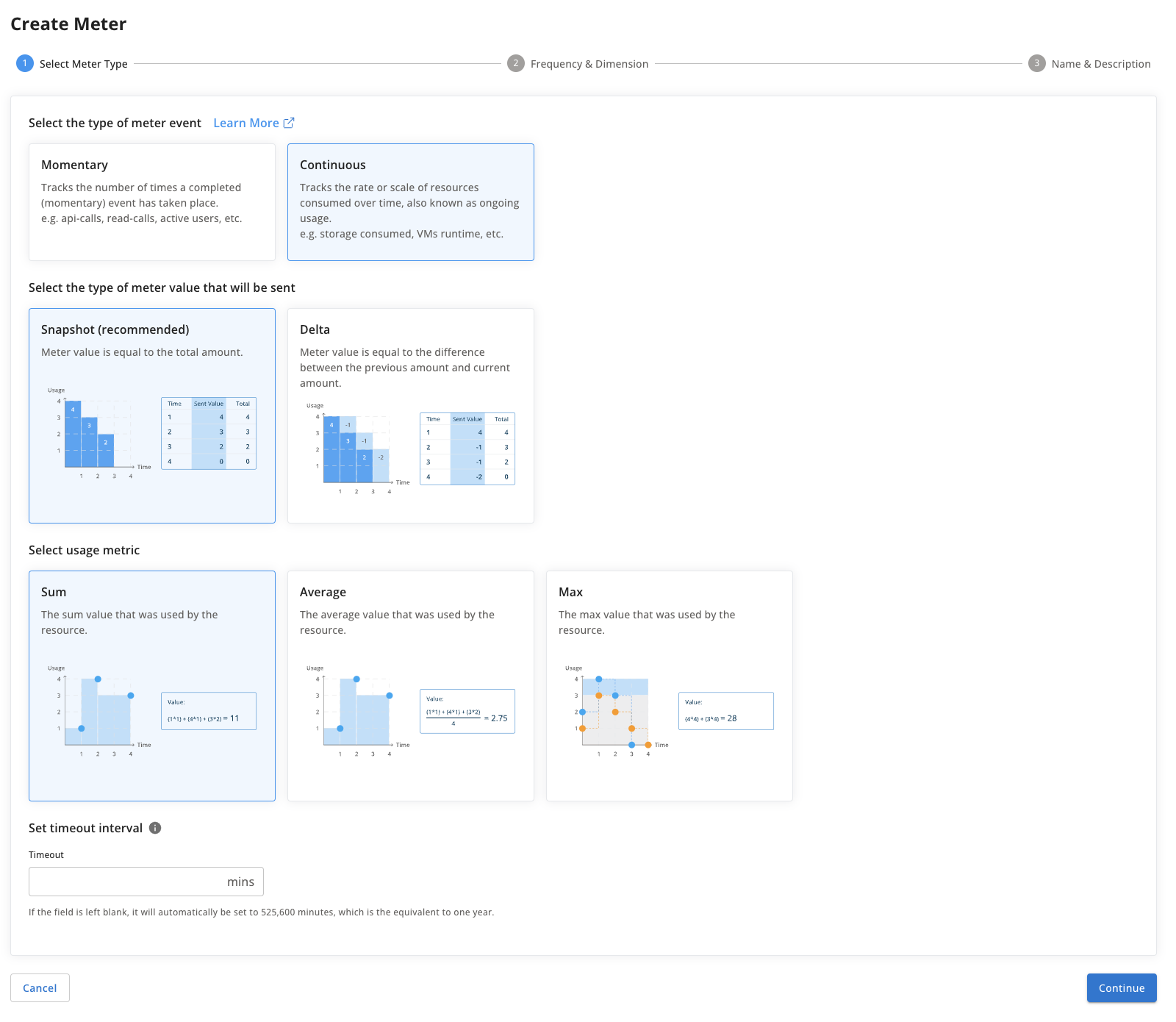Resource
A static resource is assigned to a customer over a long period of time. Examples: VM instances, active connection, etc. Another way of looking at this is resource leasing.

Meter Calculation
This provides how long a resource was leased to a customer.

Meter Value
A value of 1 marks the start of an event and 0 marks the end.

Aggregation: sum of hours

📘

Use Cases

Meter reports a value of 1 when a compute instance is started and a value of 0 when a compute instance is shut down. Get the duration of how long the VM was running in hours.

Other examples: workflow lifetime, task duration, help desk turnaround time, function duration.

👍

Example Calculation

Given:

• Meter Definition created with
• name = ComputeInstances
• eventIdDimension = clusterId
• timeout (max duration) = 4 hours

When the following events are created:

• <time(day 1, 1:10), meterName(ComputeInstances), customer(ENCOM), clusterId(1), value(1)>
• <time(day 1, 1:15), meterName(ComputeInstances), customer(ENCOM), clusterId(2), value(1)>
• <time(day 1, 1:45), meterName(ComputeInstances), customer(ENCOM), clusterId(2), value(0)>
• <time(day 1, 1:55), meterName(ComputeInstances), customer(ENCOM), clusterId(1), value(0)>
• <time(day 2, 1:00), meterName(ComputeInstances), customer(Stark Industries), clusterId(1), value(1)>
• <time(day 2, 9:00), meterName(ComputeInstances), customer(Stark Industries), clusterId(1), value(0)>
• <time(day 3, 1:15), meterName(ComputeInstances), customer(ENCOM), clusterId(4), value(1)>
• <time(day 3, 3:45), meterName(ComputeInstances), customer(ENCOM), clusterId(4), value(0)>
• <time(day 4, 23:30), meterName(ComputeInstances), customer(ENCOM), clusterId(5), value(1)>

Then:

• Query range of day 1 to day 1 -> value 1.25
• Query range of day 2 to day 2 -> value 4 # timeout exceeded
• Query range of day 3 to day 3 -> value 2.5
• Query range of day 1 to day 3 -> value ENCOM(5.25), Stark Industries(2.5)
• Query range of day 4 to day 4 -> value 0.5
• Query range of day 5 to day 5 -> value 3.5 # carried over from day 4 and timed out

## Event Duration with Hourly Average

Duration is tracked using the eventId dimension. A start event with value 1 is sent when usage begins. The count remains active until a new meter event decrements it (ie. a stop event having the same eventId dimension with value 0). The value of 1 is carried over to the next hour until the event is ended or the timeout is reached.

This meter tracks the average usage rate

This meter is created by selecting Continuous as the type of meter event, Snapshot as the type of meter value that will be sent, and Sum as the usage metric.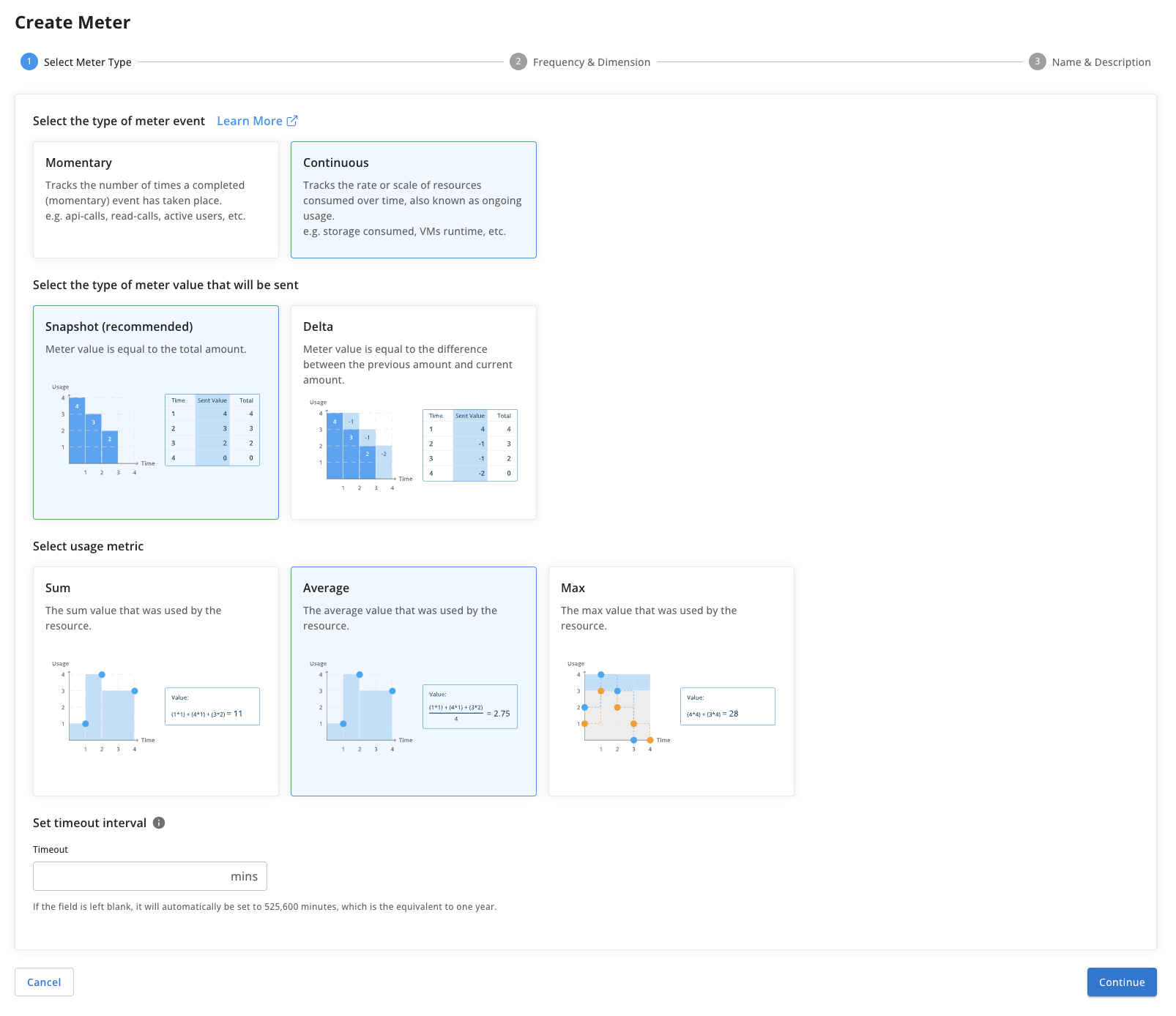Resource
A static resource is assigned to a customer over a long period of time. Examples: VM instances, active connection, etc. Another way of looking at this is resource leasing.

Meter Calculation
This provides the average usage rate over the length of time that the resource was leased to the customer.

Meter Value
The meter value for the start event is the usage rate at that time. The

Aggregation: sum of hours

## Max Usage

This meter will calculate the maximum usage rate over a given time. If no value is reported for a subsequent time interval then the previous interval will be persisted (carried forward) until a new value is received or the timeout period expires. At timeout, the value is reset to 0. The events are matched by the value of the eventId dimension.

This meter is created by selecting Continuous as the type of meter event, Snapshot as the type of meter value that will be sent, and Max as the usage metric.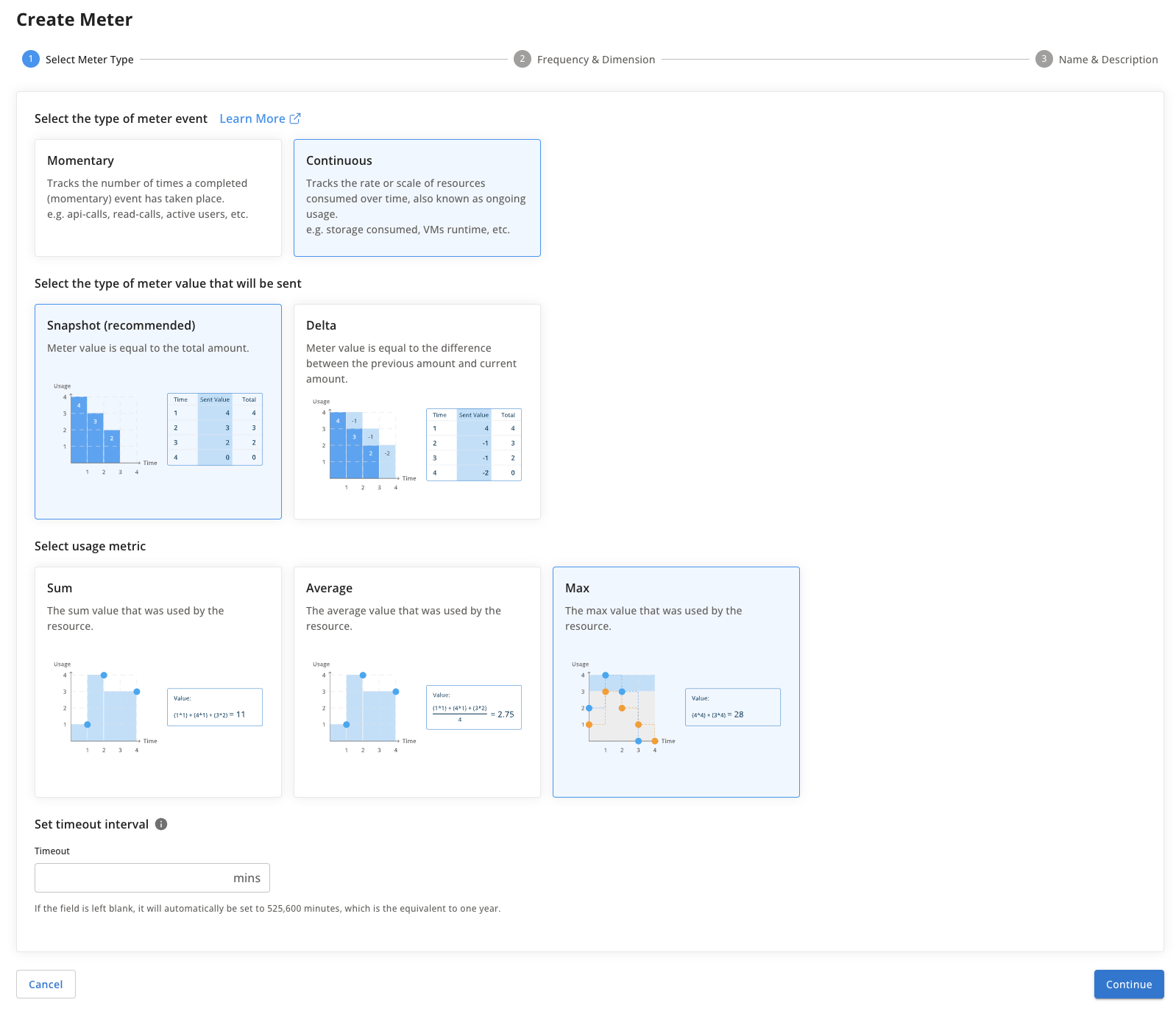Resource
A static resource is assigned to a customer over a long period of time. Examples: storage consumed, VMs used, workflow count, etc.

Meter Calculation
This meter tracks the scale or frequency a resource is being assigned to a customer over time. In other words, the meter tracks the usage rate or max utilization.

Meter Value
The observed value in its current state (aka snapshot) as opposed to the change since the previously ingested event. In other words, a snapshot is the total portion of the resource dedicated to the customer.

Aggregation: max

📘

Use Cases

Example 1: You report the size of a storage bucket every time it is changed with no set interval. Max-Persist will carry the last reported value forward. The storage bucket (e.g. AWS S3) id is the EventId. Even if there is no new event reported, you can keep the usage (and billing) carried over (since events are sent only when a change occurs, and reports the total storage used.

Example 2: You wish to meter hard drives' storage used for a server. Every hour you report each hard drive’s in-use storage. The hard drive id is the EventId. You can set the timeout to how long you want to keep on charging if there is no new event reported (e.g. 1 month).

👍

Example Calculation

Given:

• Meter Definition created with name: DataStorage
• timeout (max duration before meter value reset to 0) is 4 hours

When the following events are created:

• <time(day 1, 1:10), meterName(DataStorage), customer(Stark), value(8)>
• <time(day 1, 1:15), meterName(DataStorage), customer(Stark), value(3)>
• <time(day 1, 1:55), meterName(DataStorage), customer(Stark), value(9)>
*<time(day 1, 7:55), meterName(DataStorage), customer(Stark), value(11)>
• <time(day 2, 1:02), meterName(DataStorage), customer(ENCOM), value(6)>
• <time(day 2, 1:25), meterName(DataStorage), customer(Stark), value(4)>
• <time(day 2, 9:00), meterName(DataStorage), customer(Stark), value(1)>

Then:

• Query range for the entire usage of day 1 at 1:00 to day 1 at 2:00 -> value 9
• Query range for the entire usage of day 1 at 2:00 to day 1 at 3:00 -> value 9 (value persisted)
• Query range for the entire usage of day 1 at 6:00 to day 1 at 7:00 -> value 0 (value timed out and reset)
• Query range for usage of day 2 at 1:00 to day 2 at 2:00 of customer ‘Stark’ -> value 4
• Query range for usage of day 2 at 1:00 to day 2 at 2:00 of customer ‘ENCOM’ -> value 6
• Query range for the entire usage of day 2 at 1:00 to day 2 at 2:00 -> value (6 + 4) -> value 10

# Delta Meters

## Running Total

This meter tracks continuous events except it tracks the running total of all events assigned to a customer as opposed to reporting a time duration. The meter value can be incremented or decremented.

You need to specify the event ID dimension** in the meter definition to associate meters to an event and resource. Once the timeout period expires, the count will be reset automatically.

This meter is created by selecting Continuous as the type of meter event, Delta as the type of meter value that will be sent, and Max as the usage metric.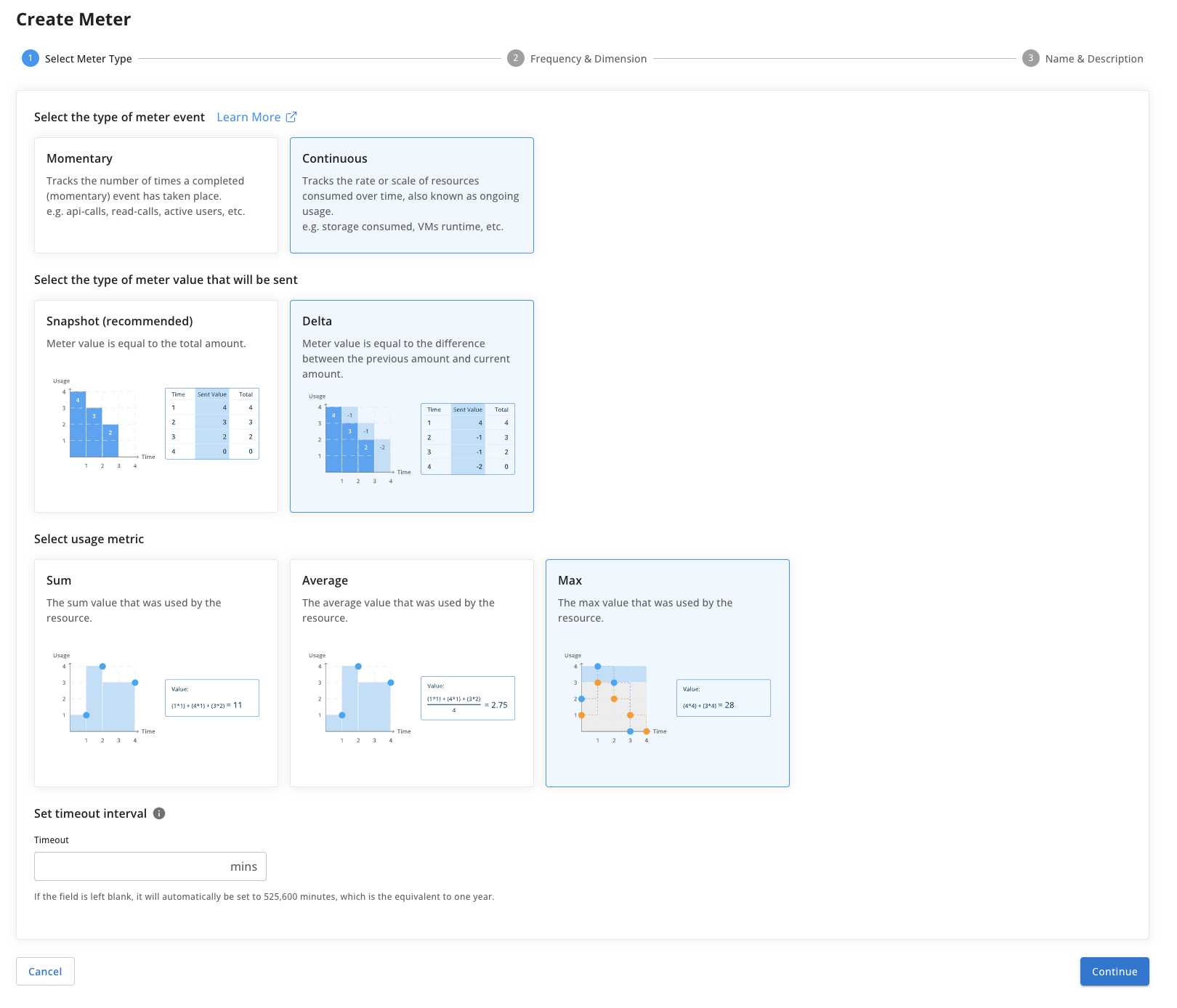Resource
A static resource is assigned to a customer over a long period of time. Examples: VMs used, active connections, etc.

Meter Calculation
This provides the number of resources assigned to a customer.

Meter Value
Overall, tracks the net current running total of instances that were up. It is an in-use counter where the delta can be an increment or decrement.

Aggregation: max

📘

Use Cases

A meter reports the active connections at the start of every hour.

Other examples: active VMs, active tickets.

👍

Example Calculation

Given:

• Meter Definition created with
• name = ActiveConnections
• eventIdDimension = instanceId
• timeout = 4 hours

When the following events are created:

• <time(day 1, 1:10), meterName(ActiveConnections), customer(ENCOM), instanceId(1), value(1)>
• <time(day 1, 1:15), meterName(ActiveConnections), customer(ENCOM), instanceId(2), value(1)>
• <time(day 1, 1:20), meterName(ActiveConnections), customer(ENCOM), instanceId(3), value(1)>
• <time(day 1, 1:30), meterName(ActiveConnections), customer(ENCOM), instanceId(1), value(-1)>
• <time(day 1, 1:45), meterName(ActiveConnections), customer(ENCOM), instanceId(2), value(-1)>
• <time(day 1, 1:50), meterName(ActiveConnections), customer(ENCOM), instanceId(3), value(-1)>
• <time(day 2, 1:00), meterName(ActiveConnections), customer(Stark Industries), instanceId(1), value(1)>
• <time(day 2, 9:00), meterName(ActiveConnections), customer(Stark Industries), instanceId(1), value(-1)> # ignored due to timeout
• <time(day 3, 1:15), meterName(ActiveConnections), customer(ENCOM), instanceId(4), value(1)>
• <time(day 3, 3:45), meterName(ActiveConnections), customer(ENCOM), instanceId(4), value(-1)>
• <time(day 4, 23:30), meterName(ActiveConnections), customer(ENCOM), instanceId(5), value(1)>

Then:

• Query range of day 1 to day 1 -> value 3
• Query range of day 2 to day 2 -> value 1
• Query range of day 3 to day 3 -> value 1
• Query range of day 1 to day 3 -> value ENCOM(4), Stark Industries(1)
• Query range of day 4 to day 4 -> value 1
• Query range of day 5 to day 5 -> value 1 # carried over from day 4 and timed out

## Resource Leasing

This is another delta meter for tracking continuous events (resource usage) such as data storage or VM runtime. In this model, rather than reporting the total usage periodically (snapshot method), the system would report whenever the usage rate changed (where the meter value is the magnitude of the change).

Note that we tend to recommend the snapshot reporting method wherever possible (i.e. Max Usage, Event Duration meters), but recognize that for some systems and use cases it is more feasible to report on the deltas.

This meter is created by selecting Continuous as the type of meter event, Delta as the type of meter value that will be sent, and Sum as the usage metric.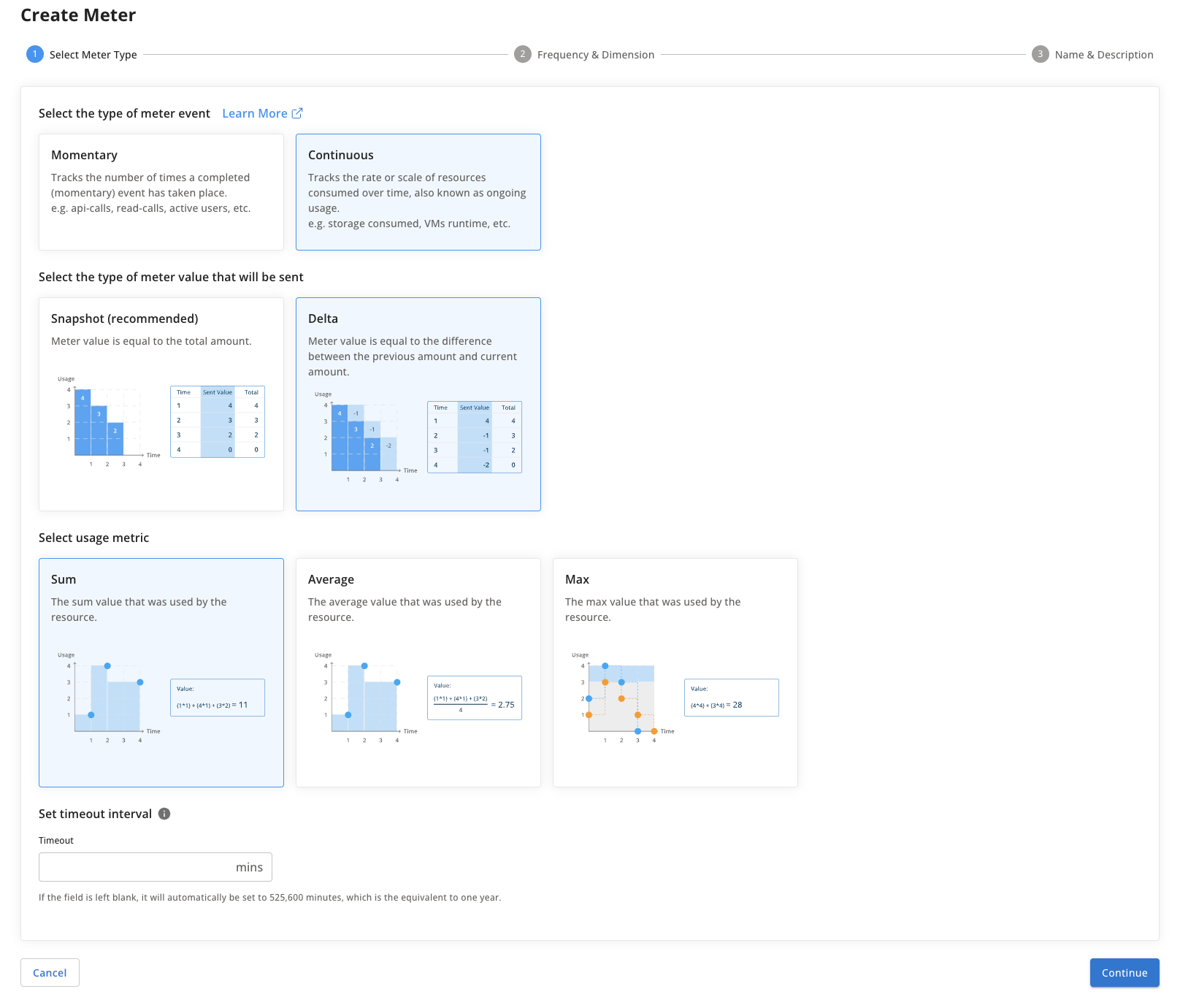Resource
A static resource is assigned to a customer over a long period of time. Examples: VMs used, active connections, etc.

Meter Calculation
This provides the change in usage rate of resources assigned to a customer.

Meter Value
Overall, tracks the net current running total of instances that were up. It is an in-use counter where the delta can be an increment or decrement.

Aggregation: sum

## Resource Leasing with Hourly Average

This is another delta meter for tracking continuous events (resource usage) such as data storage or VM runtime. In this model, rather than reporting the total usage periodically (snapshot method), the system would tracks the hourly average usage rate of a particular resource.

Note that we tend to recommend the snapshot reporting method wherever possible (i.e. Max Usage, Event Duration meters), but recognize that for some systems and use cases it is more feasible to report on the deltas.

This meter is created by selecting Continuous as the type of meter event, Delta as the type of meter value that will be sent, and Average as the usage metric.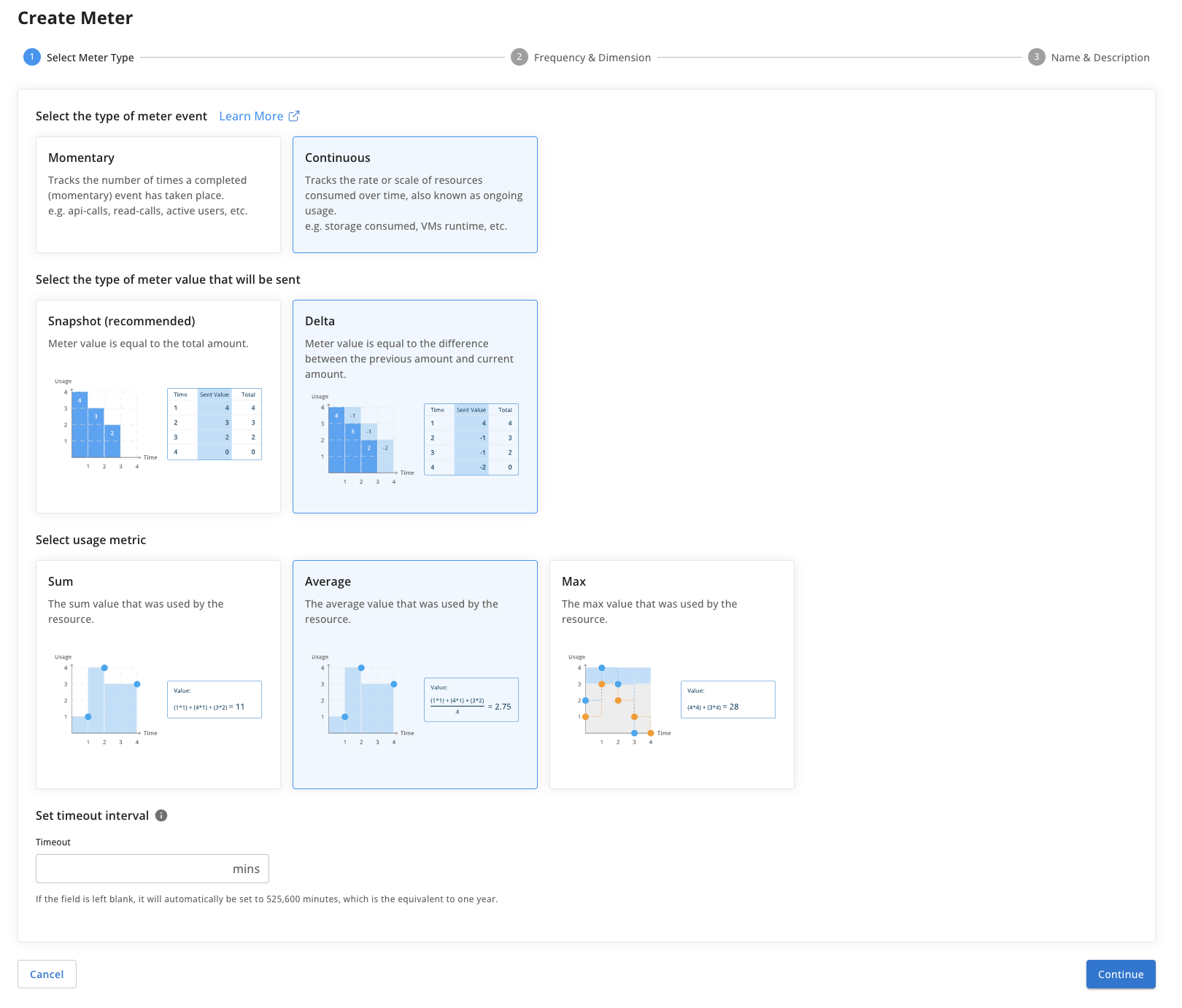Resource
A static resource is assigned to a customer over a long period of time. Examples: VMs used, active connections, etc.

Meter Calculation
This provides the hourly average change in usage rate of resources assigned to a customer.

Meter Value
Overall, tracks the average hourly usage rate for a resource. It is an in-use counter where the delta can be an increment or decrement.

Aggregation: average